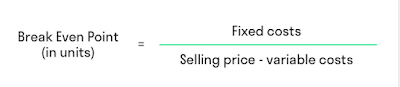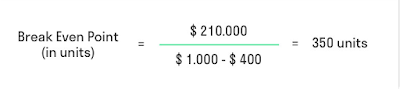## Thursday 31 August 2017

Analysis of the Break Even Point (BEP) is one of several powerful tools for managers in carrying out its operational activities. This tool helps them understand the reciprocal links between costs, volume and profit in the organization with a focus on interactions between the five elements, namely the price of the staple, volume/level of activity, the variable cost per unit, total fixed costs and the product mix for sale.

## Analysis Of Break Even Point

To analyze the Break Even Point (BEP) or breakeven noteworthy staple elements that influence, namely: cost, sales volume and selling price. The third element of the staple should not be separated because of the intertwined, where the cost of determining the selling prices, selling prices affect sales volume, the volume of sales influenced the production volume and production volume affects costs.

### Profit

A profit is the difference between more income over the burden in connection with business activities. Also called profit with income, earnings or pros is a summary of the results of operating activities NET effort into certain periods expressed in financial terms. The profit was the company's most sought after information in the money market.

Profit is the right tool to measure the achievements of the leadership and management of the company who is an indicator in the successful or whether managers. A major factor in his little big profit is income and costs.

### Selling price

Price and sales volume of mutual influence. The large number of sales volume of a product are strongly influenced by price, either for producers or for consumers. Selling price can be a net selling price or gross sale price. The fixing of the selling price of a product is very important, errors in pricing would be fatal for the financial establishments and will affect the continuity of the business.

Here are some of the methods used in the determination of the sale price:

Cost-plus pricing. Is the determination of the sale price by adding the expected profit over the full amount of the future to manufacture and market the product.
Time and material pricing. Is the determination of the sale price of the full costs plus a profit is expected, this method is used by the company car repair shop, docks and other companies that sell spare parts or repair as a complementary sales services. The volume of services is calculated based on the time it takes to serve consumer needs to be calculated so that the selling price or unit of time enjoyed by consumers.

Cost type contract pricing. Is the contract manufacturing of goods or services which buyers agree to purchase goods or services appropriate price based on the total fees actually incurred by the manufacturers coupled with the calculated profit amounting to a percentage of the total cost indeed.

### Fees

Cost or cost is an economical source of sacrifice is measured in units of money that has occurred or is likely to occur to achieve a particular goal. This fee is not yet running out of life and are classified as assets to be included in the balance sheet. While burden or expense is a cost that has given the benefits and has now been discharged. The fees have not enjoyed that can provide benefits in the forthcoming period are classified as treasure. These costs are incorporated into the Profit and loss, as a deduction from income.

Based on its nature (by nature), the cost can be classified into three types:

Fixed costs (Fixed Cost = FC).

Fixed costs are costs that total amount remains constant is not influenced changes in the volume of production in the period and a certain level. But at the cost of these fixed costs unit (unit cost) will change inversely proportional to changes in the volume of production. The higher the production volume, the lower the unit cost. Conversely, the lower the volume of the higher production cost per unit. The types of costs that are classified as fixed costs among others are: shrinking machine, building depreciation, rent, insurance company assets, a fixed monthly salary of employees anyway.

Variable Costs. Variable costs are costs that total amount of change is proportional (proportional) in accordance with changes in the volume of production. The greater the volume of production increasingly greater amount of total variable costs incurred. Instead the smaller the volume of the production of increasingly small amount from the total cost. The type of the variable costs include: costs of raw materials, direct labor costs, the cost of electric power of the machine, and so on.
Semi Variable Cost. The cost of semi-private variable is the sum of the total cost will be changed according to changes in the volume of production, but the changes are disproportionate. In automatic analysis-break-even, the cost should be grouped into two groups namely fixed cost and variable cost.

## Method Of Calculation Of Break Even Point

Break even point can generally be calculated by three methods i.e. method of equations, and the method of contribution margin method of graphics. The third method is essentially the approach that has the end result is the same, but the methods have the third difference in shapes and variation from the equation of income statement contributions.

### Method Equation

Method of equation (equation method) is a method which is based on the approach of the income statement. Determination of break even or the break-even equation technique done by basing on the equation of revenue equal to cost plus profit.

Profit is calculated by the following formula:

Description:
y = profit
c = selling price unity
x = the number of products sold
b = variable cost the Union
a = fixed costs

As for formulas Break Even Point (BEP) is the equation method as follows:Break Even Point Equation formula in DollarBreak Even Point Equation formula in unit

### Method of Contribution Units

Unit Contribution method is the method of variation equation. Any unit or units of product sold will generate a certain amount of the contribution margin will offset the costs anyway. Unit contribution method is the method by which the shortcut should be known the value of the contribution margin.
Contribution Margin
is the result of revenue reduction from the sale with the variable costs.

### Graphic Method

Graphics breakeven (BEP) has some important things during the sale price exceeds the variable cost (contributors positive margin), then more sales will benefit the company, either by increasing the profits or reduce losses.

Graph of cost-volume-profit (cost volume profit graph) describes the relationship between costs, volume and profit. To get a more detailed picture of the need created graphs with two separate lines, namely the line of the total revenue and total cost. Analysis of the breakeven or a break even point (BEP) and graphical methods are described on the curve as shown below:

Description:

• Flat-axis (x axis) declared sales volume which can be expressed in units of quantity or sales revenue is in rupiah.
• Vertical axis (y axis) stated sales revenue and the cost in rupiah.
• Break even (BEP) is located at the intersection of the line with the line cost of sales income. If the intersection point of line drawn straight to the x axis, will note the achievement of breakeven based on sales volume. If from the breakeven line drawn perpendicular to the y-axis, will note the achievement of breakeven sales based on income.
• The area to the left of break-even, i.e., the total cost of the line between areas with sales income is a loss, because the sales revenue is lower than the total costs. Whereas the area to the right of break-even i.e., the areas between the lines of sales revenue with the total cost of the line is profit, due to higher sales revenues from total costs.